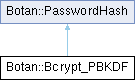Botan 2.19.1 Crypto and TLS for C&
Botan::Bcrypt_PBKDF Class Referencefinal

`#include <bcrypt_pbkdf.h>`

Inheritance diagram for Botan::Bcrypt_PBKDF:## Public Member Functions

Bcrypt_PBKDF (const Bcrypt_PBKDF &other)=default

Bcrypt_PBKDF (size_t iterations)

void derive_key (uint8_t out[], size_t out_len, const char *password, size_t password_len, const uint8_t salt[], size_t salt_len) const override

size_t iterations () const override

size_t memory_param () const override

Bcrypt_PBKDFoperator= (const Bcrypt_PBKDF &)=default

size_t parallelism () const override

std::string to_string () const override

size_t total_memory_usage () const override

## Detailed Description

Bcrypt-PBKDF key derivation function

Definition at line 19 of file bcrypt_pbkdf.h.

## ◆ Bcrypt_PBKDF() [1/2]

 Botan::Bcrypt_PBKDF::Bcrypt_PBKDF ( size_t iterations )
inline

Definition at line 22 of file bcrypt_pbkdf.h.

22: m_iterations(iterations) {}
size_t iterations() const override
Definition: bcrypt_pbkdf.h:36

## ◆ Bcrypt_PBKDF() [2/2]

 Botan::Bcrypt_PBKDF::Bcrypt_PBKDF ( const Bcrypt_PBKDF & other )
default

## ◆ derive_key()

 void Botan::Bcrypt_PBKDF::derive_key ( uint8_t out[], size_t out_len, const char * password, size_t password_len, const uint8_t salt[], size_t salt_len ) const
overridevirtual

Derive a new key under the current Bcrypt-PBKDF parameter set

Definition at line 15 of file bcrypt_pbkdf.cpp.

18 {
19 bcrypt_pbkdf(output, output_len,
21 salt, salt_len,
22 m_iterations);
23 }
void bcrypt_pbkdf(uint8_t output[], size_t output_len, const char *pass, size_t pass_len, const uint8_t salt[], size_t salt_len, size_t rounds)
size_t salt_len
Definition: x509_obj.cpp:25

References Botan::bcrypt_pbkdf(), and salt_len.

## ◆ iterations()

 size_t Botan::Bcrypt_PBKDF::iterations ( ) const
inlineoverridevirtual

Most password hashes have some notion of iterations.

Definition at line 36 of file bcrypt_pbkdf.h.

36{ return m_iterations; }

## ◆ memory_param()

 size_t Botan::Bcrypt_PBKDF::memory_param ( ) const
inlineoverridevirtual

Some password hashing algorithms have a parameter which controls how much memory is used. If not supported by some algorithm, returns 0.

Definition at line 40 of file bcrypt_pbkdf.h.

40{ return 0; }

## ◆ operator=()

 Bcrypt_PBKDF & Botan::Bcrypt_PBKDF::operator= ( const Bcrypt_PBKDF & )
default

## ◆ parallelism()

 size_t Botan::Bcrypt_PBKDF::parallelism ( ) const
inlineoverridevirtual

Some password hashing algorithms have a parallelism parameter. If the algorithm does not support this notion, then the function returns zero. This allows distinguishing between a password hash which just does not support parallel operation, vs one that does support parallel operation but which has been configured to use a single lane.

Definition at line 38 of file bcrypt_pbkdf.h.

38{ return 0; }

## ◆ to_string()

 std::string Botan::Bcrypt_PBKDF::to_string ( ) const
overridevirtual

Definition at line 25 of file bcrypt_pbkdf.cpp.

26 {
27 return "Bcrypt-PBKDF(" + std::to_string(m_iterations) + ")";
28 }
std::string to_string(const BER_Object &obj)
Definition: asn1_obj.cpp:213

References Botan::ASN1::to_string().

## ◆ total_memory_usage()

 size_t Botan::Bcrypt_PBKDF::total_memory_usage ( ) const
inlineoverridevirtual

Returns an estimate of the total memory usage required to perform this key derivation.

If this algorithm uses a small and constant amount of memory, with no effort made towards being memory hard, this function returns 0.